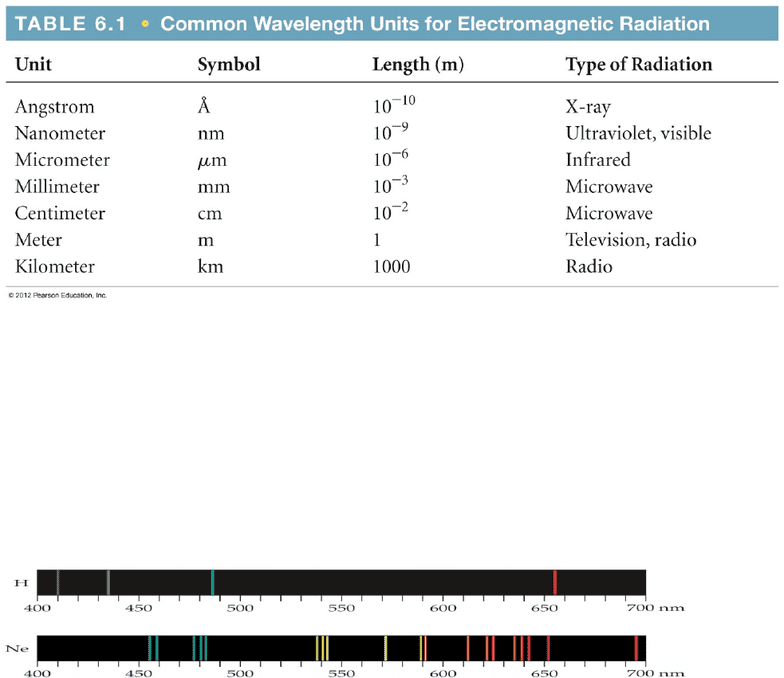# CHEM104 Study Guide - Final Guide: Enthalpy, Heat Capacity, Internal Energy

119 views16 pages
School
University of Regina
Department
Chemistry
Course
CHEM104
ProfessorChemistry 104
Thermochemistry
Energy is the ability to do work (
w=F × D
) or transfer heat. Energy can also be transferred as heat. The energy used to cause the
temperature of an object to rise where the heat will flow from the warmer object to the cooler object
Units
The unit we will be using is the joule where
1joule=kg ∙m2
s2
or
1calorie=4.184 joules
System versus Surrounding
System includes the molecules we want to study. Surrounding is everything else!
1ST LAW OF THERMOCHEMISTY
E of system= E surroundings
Energy cannot be created or destroyed. The internal energy of a system is the sum of all kinetic and potential energy of
all components of the system, called Energy.
Change in Energy is the final energy minus the initial energy
∆ E=q+w
Positive/ Negative E Q W
+ Net gain Gains heat Work done on
- Net loss Loses heat Work done by
Exchange of Heat
Exothermic reactions mean heat is released, and the enthalpy is positive
Endothermic reactions mean heat is absorbed, and the enthalpy is negative
Enthalpy
H=E+Pv
∆ H=∆ E+P∆ V
Gain of energy =positive
Energy
E initial
Loss of energy =
Negative Energy
E final
Unlock document

This preview shows pages 1-3 of the document.
Unlock all 16 pages and 3 million more documents.The system only changes at constant pressure
Work done by gas
Enthalpy of Reaction
The change in Enthalpy
∆ H= H products∆ Hreactants
1. H for a reaction in the forward direction is equal but opposite to the reaction occurring in the back direction
2. H for a reaction depends on the state of the products and state of the reactants
Calorimetry
Calorimetry measures heat flow. The instrument used to measure heat flow (q) is called a calorimeter
∆ Hreaction=qreaction
q=Csm∆ t=Cmn ∆ t
(m=mass, n=moles)
Heat capacity (Cm) is the amount of energy required to the increase the temperature of a substance by one Kelvin or one degree Celsius for one mole
of substance
Cm=Joules
mole × ∆ t ° C
Specific heat capacity (Cs) is the amount of energy required to increase the temperature of one gram of a substance by one kelvin or one degree
Celsius.
Calculation of H
We can estimate H using published H values and the properties of enthalpy
Hess’s Law
If a reaction is carried out in a series of steps H for the overall reaction will be equal to the sum of the enthalpy change for the individual steps
Enthalpies of Formation
An enthalpy of formation, Hf is defined as the enthalpy change for the reaction in which a compound is made from its constituent elements in their
elemental form
Standard enthalpies of formation
Are measured under standard conditions (25ºC and 1.00 atm pressure) This is called a formation reaction
Unlock document

This preview shows pages 1-3 of the document.
Unlock all 16 pages and 3 million more documents.Electronic Structure of an Atom
Light and Matter
Light has wave like properties and is a self-propagating wave with electric and magnetic components
Waves
is the symbol denoting wavelength between two pointsλ
v is the symbol denoting frequency, the number of waves per period of time
if the time associated with the lines to the left is one second then the frequencies would be 2s-1 and 4s-1
All electromagnetic radiation travels at the speed of light (c)= 3.00×108m/s
c=λv
The longer the wavelength, the smaller the frequency
The Photoelectric Effect
Electrons are emitted from metals when they absorb energy from light. At certain lower frequencies, electrons are not emitted, regardless the intensity of
light.
The Energy of a photon E=hv where h=6.626x 1034 J ∙ s
Line Spectra &The Bohr Model
Atomic Emissions
Only a line spectrum of discrete wavelengths is observed where each element has a unique line spectrum
Unlock document

This preview shows pages 1-3 of the document.
Unlock all 16 pages and 3 million more documents.